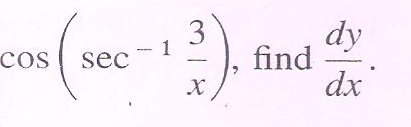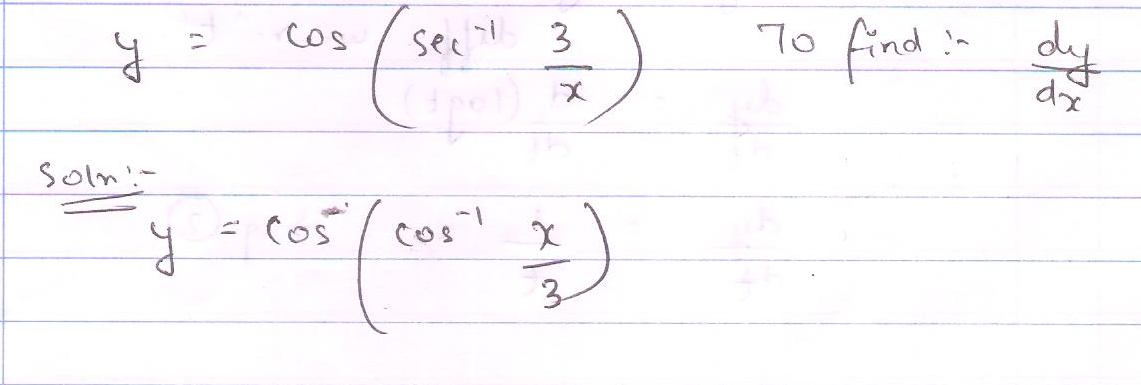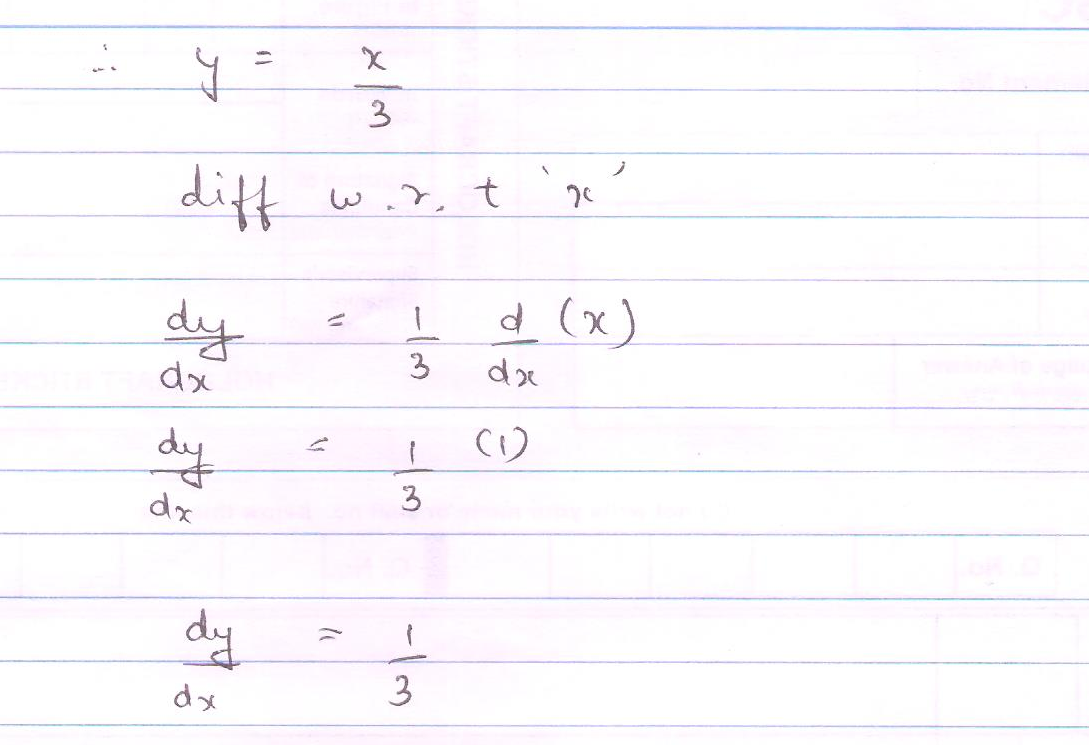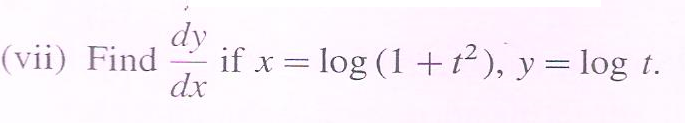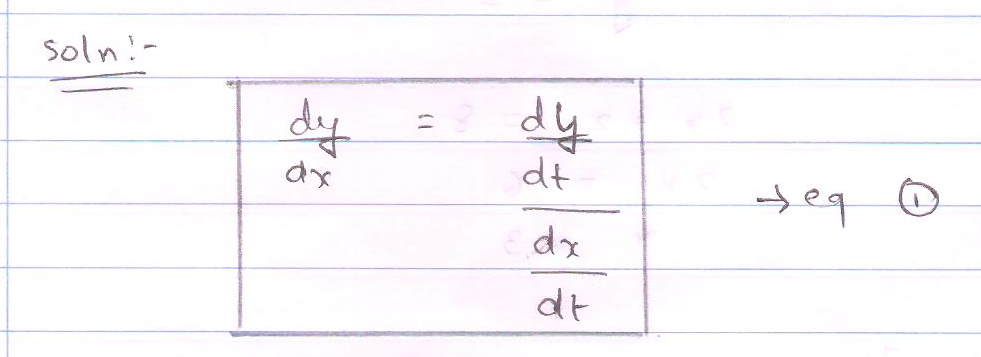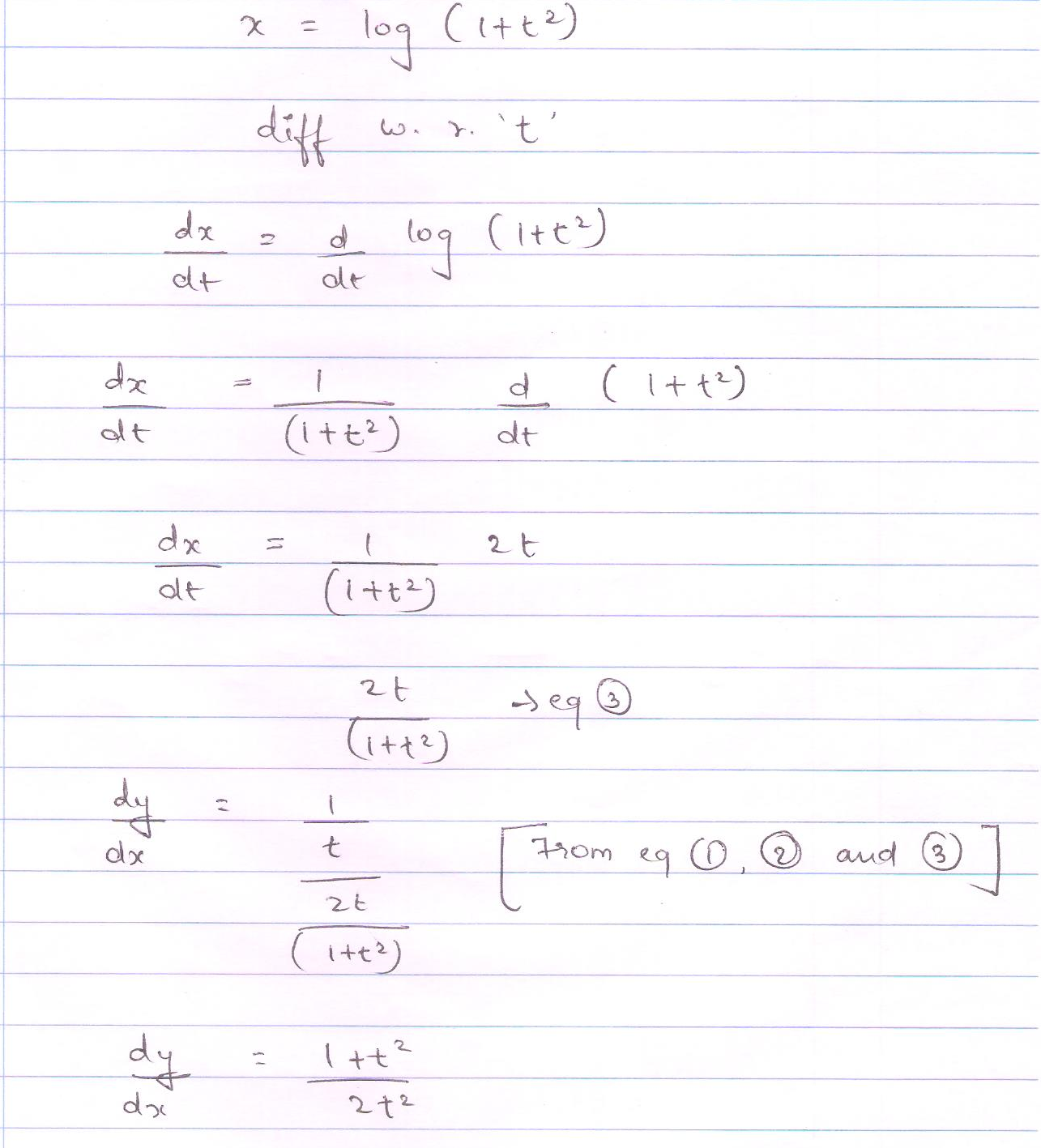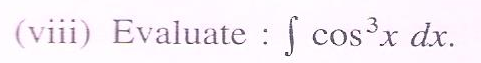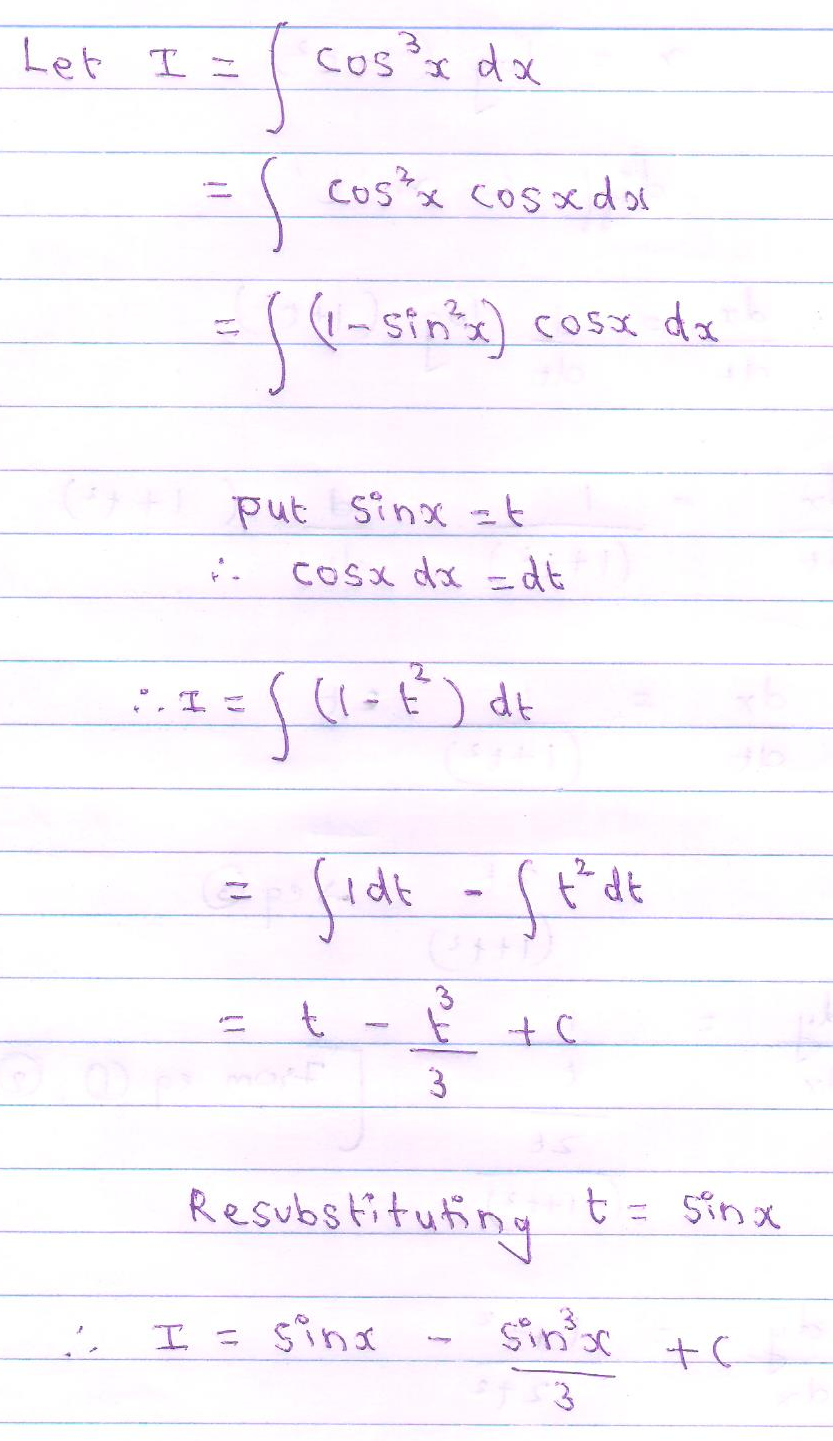### HSC COMMERCE PRACTICE PAPER WITH SOLUTION

HSC COMMERCE PRACTICE PAPER WITH SOLUTION

Q1. Attempt any Six of the Following: 

(i) Write the following statements in symbolic form:
1. I like reading but not singing.
2. Even though it is not cloudy, it is still raining.

Ans.
1. Let p = I like reading
q = I like singing

Symbolic form  = (p Λ ~ q)

b. Let p = It is cloudy
q = It is still raining

Symbolic form = ~ p Λ q.

(ii) If A =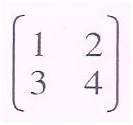and X is a 2 × 2 matrix such that AX = I then find X.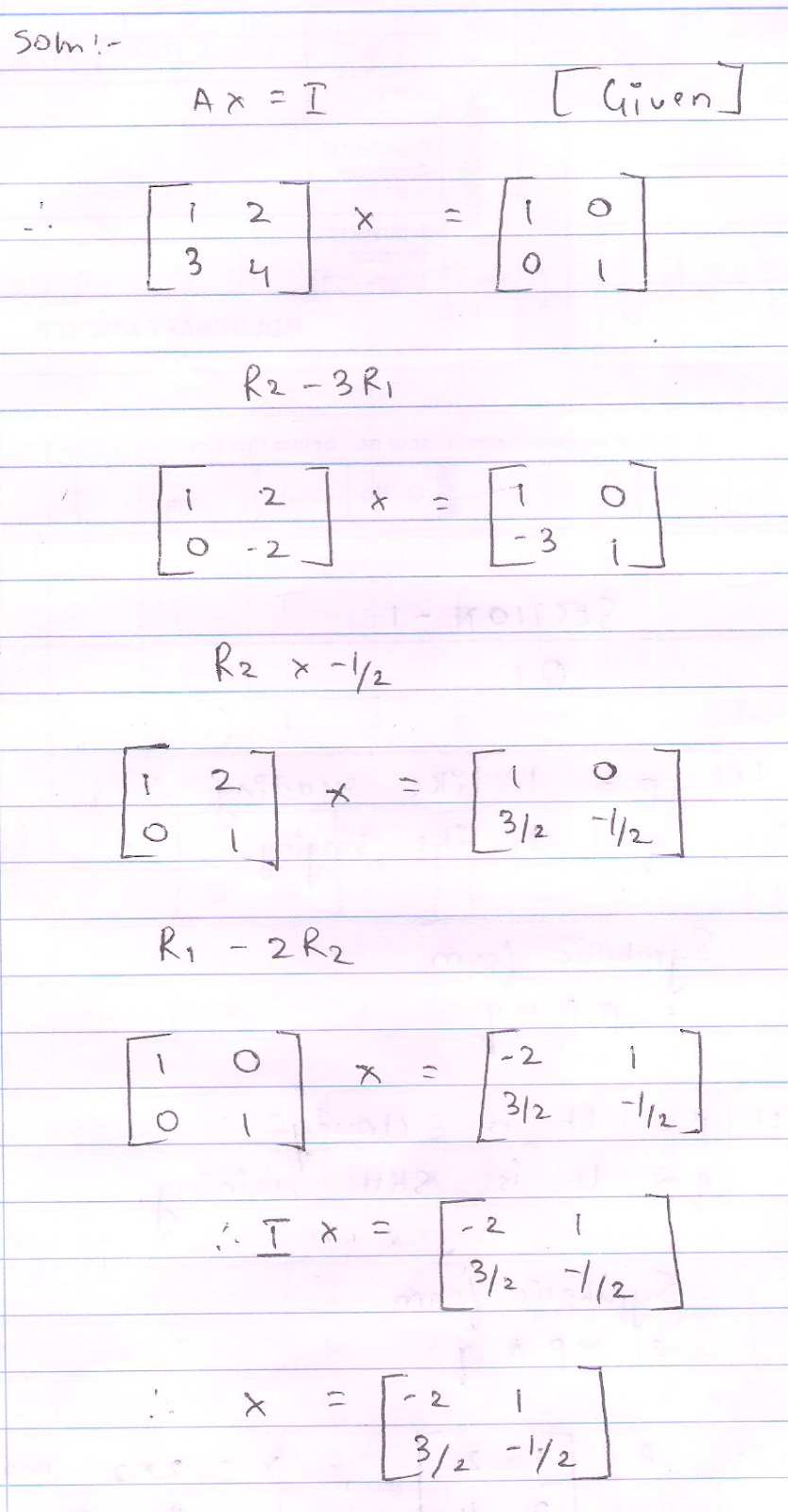(iii) Find k, if the function f is continuous at x = 0, where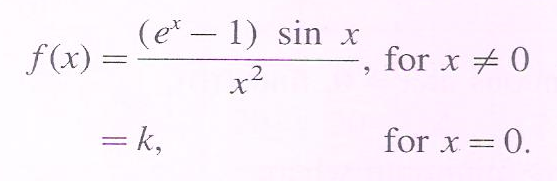Solution :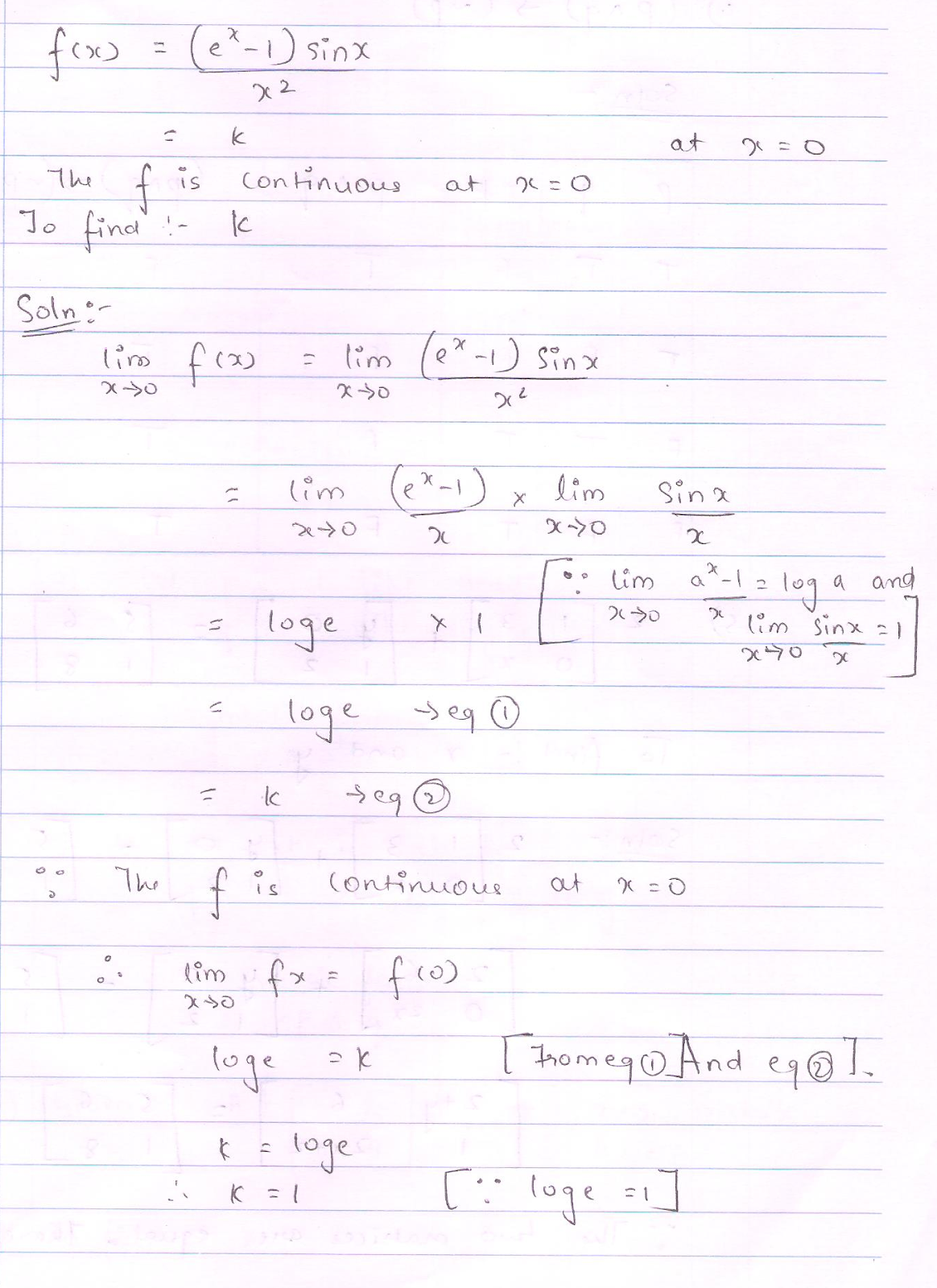(iv) Prepare the truth table of the following statement pattern: (p Λ q)  ---->  ( ~ p)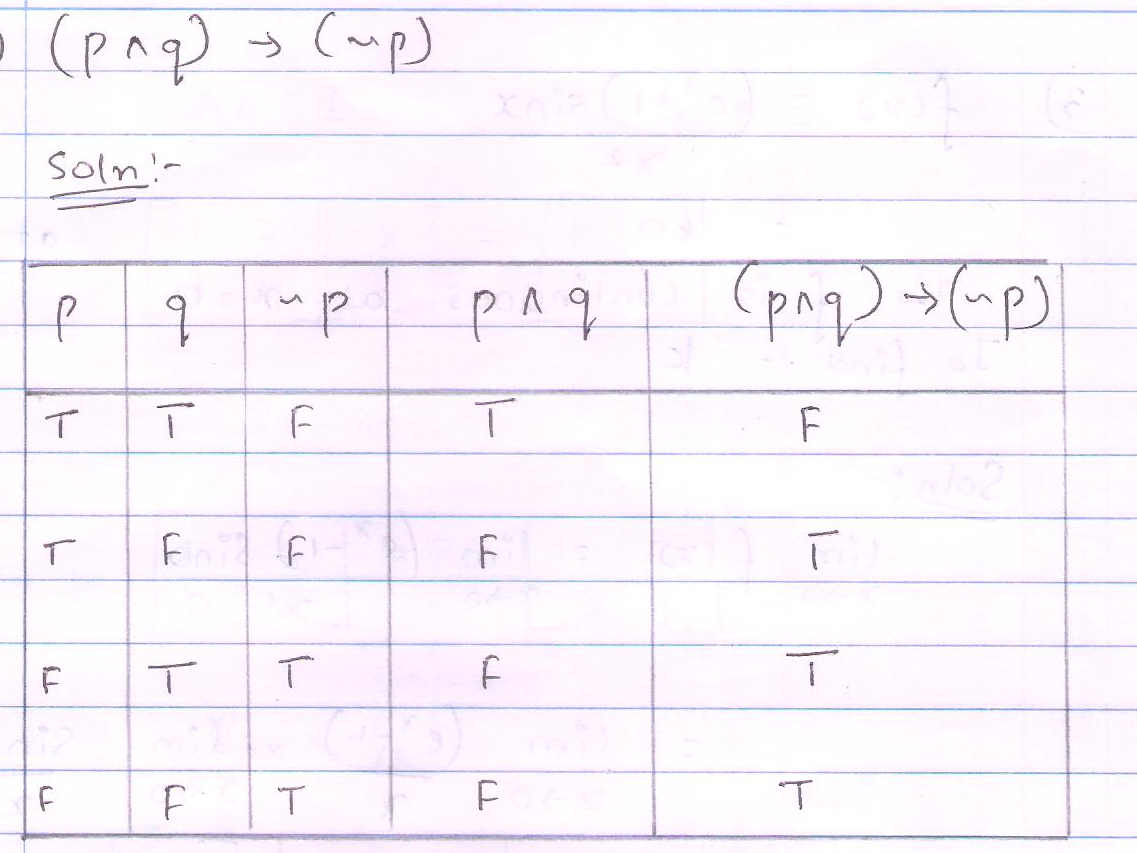v. Find the values of x and y if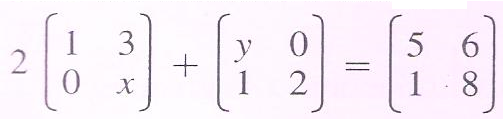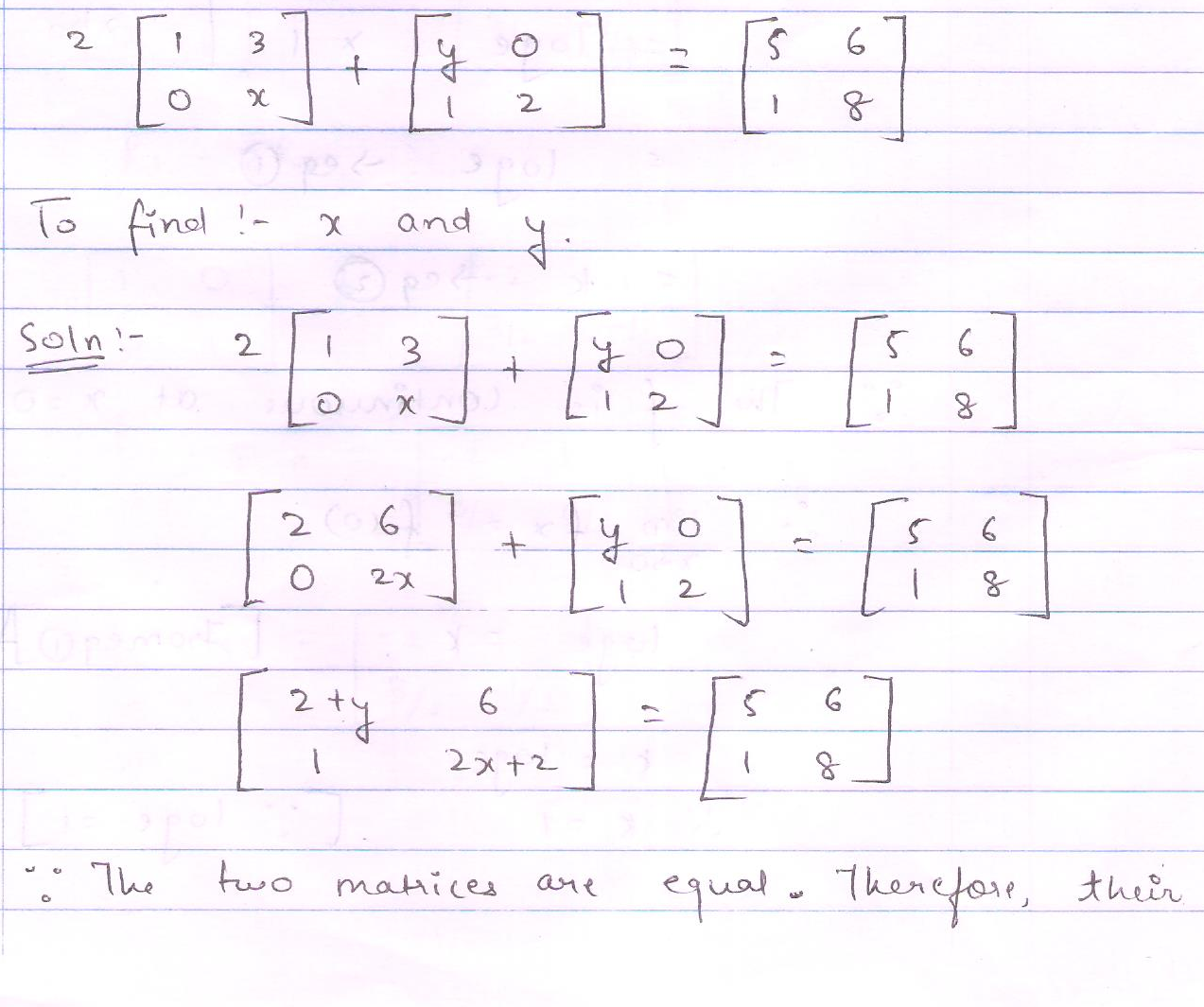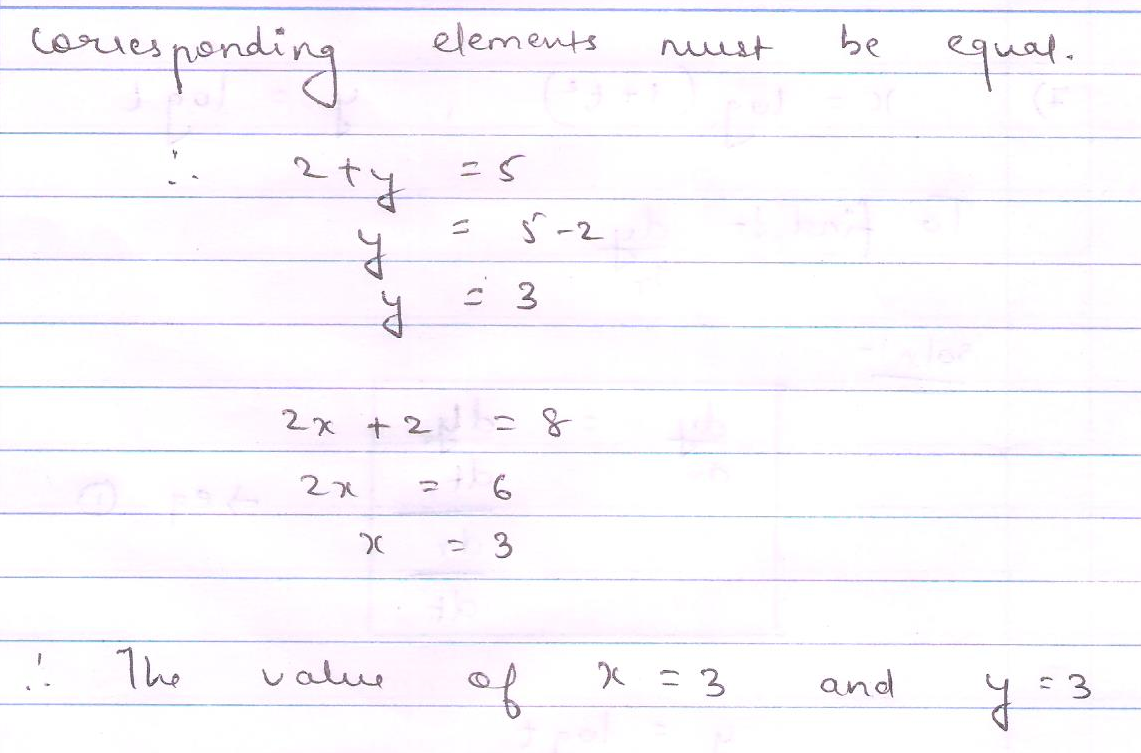(vi) If y =